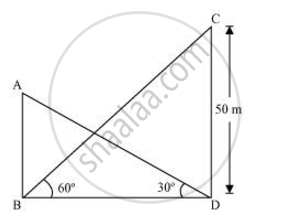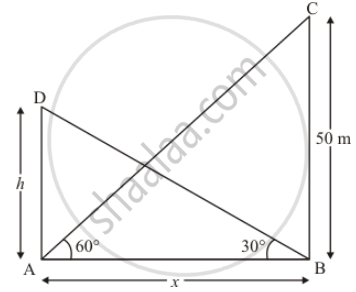Share

# The angle of elevation of the top of a building from the foot of the tower is 30° and the angle of elevation of the top of the tower from the foot of the building is 60°. If the tower is 50 m high, find the height of the building - CBSE Class 10 - Mathematics

#### Questions

The angle of elevation of the top of a building from the foot of the tower is 30° and the angle of elevation of the top of the tower from the foot of the building is 60°. If the tower is 50 m high, find the height of the building

The angle of elevation of the top of the building from the foot of the tower is 30° and the angle of the top of the tower from the foot of the building is 60°. If the tower is 50 m high, find the height of the building.

#### Solution 1Let AB be the building and CD be the tower.

In ΔCDB,

CB/BD = tan 60º

50/(BD) = sqrt3

BD = 50/sqrt3

In ΔABD,

(AB)/(BD) = tan 30º

AB = 50/sqrt3 xx 1/sqrt3 = 50/3 = 16 2/3

Therefore, the height of the building is 16 2/3 m

#### Solution 2

Let AD be the building of height h m. and an angle of elevation of the top of building from the foot of the tower is 30° and an angle of the top of the tower from the foot of building is 60°.

Let AD = hAB = x and BC = 50 and ∠DBA = 30°, ∠CAB = 60°So we use trigonometric ratios.

In a triangle ABC

=> tan 60^2 = 50/x

=> sqrt3 = 50/x

=> x = 50/sqrt3

Again in a triangle ABD

=> tan 30° = (AD)/(AB)

=> 1/sqrt3 xx h/x

=> h = x/sqrt3

=> h = 50/(sqrt3 xx sqrt3)

=> h = 50/3

Hene the height of building is  50/3 m

Is there an error in this question or solution?

#### APPEARS IN

NCERT Solution for Mathematics Textbook for Class 10 (2019 to Current)
Chapter 9: Some Applications of Trigonometry
Ex. 9.10 | Q: 9 | Page no. 204
NCERT Solution for Mathematics Textbook for Class 10 (2018 to Current)
Chapter 9: Some Applications of Trigonometry
Ex. 9.10 | Q: 9 | Page no. 204

#### Video TutorialsVIEW ALL 

Solution The angle of elevation of the top of a building from the foot of the tower is 30° and the angle of elevation of the top of the tower from the foot of the building is 60°. If the tower is 50 m high, find the height of the building Concept: Heights and Distances.
S